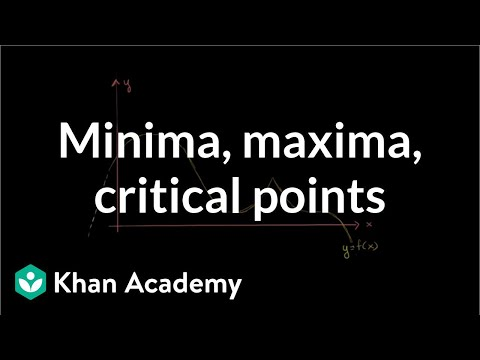### Calculus: Derivative applications

Free Closed [?]Minima, maxima, and critical points. Rates of change. Optimization. Rates of change. L'Hopital's rule. Mean value theorem.
Minima, maxima and critical points. Testing critical points for local extrema. Identifying minima and maxima for x^3 - 12x - 5. Concavity, concave upwards and concave downwards intervals. Recognizing concavity exercise. Recognizing concavity. Inflection points. Graphing using derivatives. Another example graphing with derivatives. Minimizing sum of squares. Optimizing box volume graphically. Optimizing box volume analytically. Optimizing profit at a shoe factory. Minimizing the cost of a storage container. Expression for combined area of triangle and square. Minimizing combined area. Rates of change between radius and area of circle. Rate of change of balloon height. Related rates of water pouring into cone. Falling ladder related rates. Rate of change of distance between approaching cars. Speed of shadow of diving bird. Mean Value Theorem. Introduction to L'H

Categories:

# Reviews

-- no reviews yet -- make the first review

# Alternatives All alternatives to all Khan Academy courses

-- no alternatives found for the course --
If you know any alternatives, please let us know.

# Prerequisites

-- no prerequsites found for the course --
If you can suggest any prerequisite, please let us know.

# Paths

No Paths inclusing the course. You can build and share a path with this course included.

# Certification Exams

-- there are no exams to get certification after this course --
If your company does certification for those who completed this course then register your company as certification vendor and add your exams to the Exams Directory.

# Similar courses

Courses related to the course subject

### Calculus I (Differential Calculus)

Videos on a first course in calculus (Differential Calculus).

### Calculus II (Integral Calculus)

Videos on a second course in calculus (Integral Calculus).

### Calculus One

Calculus One is a first introduction to differential and integral calculus, emphasizing engaging examples from everyday life.

Details:

### Calculus: AP Calculus practice questions

Sample questions from the A.P. Calculus AB and BC exams (both multiple choice and free answer). 2011 Calculus AB Free Response #1a…

### Calculus: Derivative applications

Minima, maxima, and critical points. Rates of change. Optimization. Rates of change. L'Hopital's rule. Mean value theo…

### Calculus: Divergence theorem

Divergence theorem intuition. Divergence theorem examples and proofs. Types of regions in 3D. 3-D Divergence Theorem Intuition.

### Calculus: Double and triple integrals

Volume under a surface with double integrals. Triple integrals as well. Double Integral 1. Double Integrals 2. Double Integrals 3.

### Calculus: Indefinite and definite integrals

Indefinite integral as anti-derivative. Definite integral as area under a curve. Integration by parts. U-substitution. Trig su…

### Calculus: Limits

Limit introduction, squeeze theorem, and epsilon-delta definition of limits. Introduction to limits. Limit at a point of discontin…

### Calculus: Line integrals and Green's theorem

Line integral of scalar and vector-valued functions. Green's theorem and 2-D divergence theorem. Introduction to the Line In…

Let us know when you did the course Calculus: Derivative applications.

 Started on: Completed on: Your grade (if any):Comments:Add the course Calculus: Derivative applications to My Personal Education Path.

 Start the course on: Duration of study: 1 Week 2 Weeks 3 Weeks 4 Weeks 5 Weeks 6 Weeks 7 Weeks 8 Weeks 10 Weeks 12 Weeks 16 Weeks Notes: